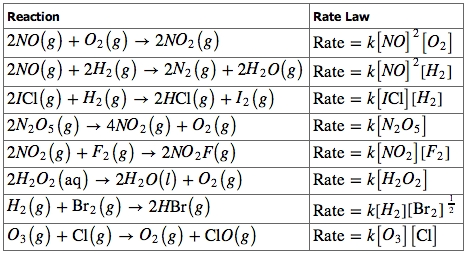## The Rate Law

#### Learning Objective

• Produce rate equations for elementary reactions

#### Key Points

• For a generic reaction $aA + bB \rightarrow C$ with no intermediate steps in its reaction mechanism (that is, an elementary reaction), the rate is given by: $r=k[A]^{x}[B]^{y}$ .
• For elementary reactions, the rate equation can be derived from first principles using collision theory.
• The rate equation of a reaction with a multi-step mechanism cannot, in general, be deduced from the stoichiometric coefficients of the overall reaction; it must be determined experimentally.

#### Term

• Rate lawAn equation relating the rate of a chemical reaction to the concentrations or partial pressures of the reactants.

The rate law for a chemical reaction is an equation that relates the reaction rate with the concentrations or partial pressures of the reactants. For the general reaction$aA + bB \rightarrow C$ with no intermediate steps in its reaction mechanism, meaning that it is an elementary reaction, the rate law is given by:

$r=k[A]^{x}[B]^{y}$

In this equation, [A] and [B] express the concentrations of A and B, respectively, in units of moles per liter. The exponents x and y vary for each reaction, and they must be determined experimentally; they are not related to the stoichiometric coefficients of the chemical equation. Lastly, k is known as the rate constant of the reaction. The value of this coefficient k will vary with conditions that affect reaction rate, such as temperature, pressure, surface area, etc. A smaller rate constant indicates a slower reaction, while a larger rate constant indicates a faster reaction.Rate laws for various reactionsA variety of reaction orders are observed. Note that the reaction order is unrelated to the stoichiometry of the reactions; it must be determined experimentally.

## Reaction Order

To reiterate, the exponents x and y are not derived from the balanced chemical equation, and the rate law of a reaction must be determined experimentally. These exponents may be either integers or fractions, and the sum of these exponents is known as the overall reaction order. A reaction can also be described in terms of the order of each reactant. For example, the rate law $Rate=k[NO]^2[O_2]$ describes a reaction which is second-order in nitric oxide, first-order in oxygen, and third-order overall. This is because the value of x is 2, and the value of y is 1, and 2+1=3.

## Example 1

A certain rate law is given as $Rate=k[H_2][Br_2]^\frac{1}{2}$. What is the reaction order?

$x=1,\;y=\frac{1}{2}$

$reaction\;order=x+y=1+\frac{1}{2}=\frac{3}{2}$

The reaction is first-order in hydrogen, one-half-order in bromine, and $\frac{3}{2}$-order overall.

## Example 2

The reaction between nitric oxide and ozone, $NO(g) + O_3(g)\rightarrow NO_2(g) + O_2(g)$ , is first order in both nitric oxide and ozone. The rate law equation for this reaction is: $Rate = k[NO]^{1}[O_{3}]^{1}$. The overall order of the reaction is 1 + 1 = 2.